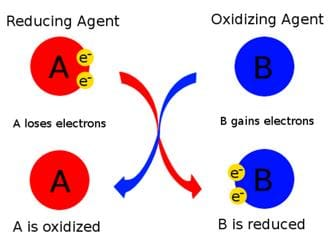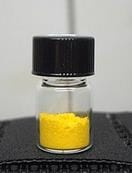# Test: Chemical Reactions & Equations

## 25 Questions MCQ Test General Science(Prelims) by IRS Divey Sethi | Test: Chemical Reactions & Equations

Description
Attempt Test: Chemical Reactions & Equations | 25 questions in 25 minutes | Mock test for Class 10 preparation | Free important questions MCQ to study General Science(Prelims) by IRS Divey Sethi for Class 10 Exam | Download free PDF with solutions
QUESTION: 1

### Which of the following is a combination reaction?

Solution:

Balanced Equation:
H2 + Cl2 → 2HCl2

The above reaction is an example of combination reaction because two different elements are combining to form a single compound.

QUESTION: 2

### Which of the following represent a double displacement reaction?

Solution:

It is a double displacement reaction. Na will displace Ag and combine with NO3 to form NaNO3. Ag will then combine with Cl and form AgCl. So the products of the reaction are AgCl and NaNO3.

QUESTION: 3

### Which of the following is a displacement reaction?

Solution:

Fe + CuSo4 → FeSO4 + Cu
is displacement reaction.
In these reaction iron (Fe) displace copper (Cu)
Hence, it is a displacement type of reaction.

(a) is a decomposition reaction.
(b) and (d) are neutralisation reactions.

QUESTION: 4

The reaction H2 + Cl2 → 2HCl is a :

Solution:

Balanced equation:
H2 + 2Cl2 → 2HCl2

The above reaction is an example of combination reaction.

QUESTION: 5

Which of the following is a decomposition reaction?

Solution:

A decomposition reaction occurs when one reactant breaks down into two or more products. Examples of decomposition reactions include the breakdown of hydrogen peroxide to water and oxygen, and the breakdown of water to hydrogen and oxygen.
2KClO3 → 2KCl + 3O2

QUESTION: 6

Which of the following statement is incorrect?

Solution:

An oxidizing agent oxidizes other substances and gains electrons; therefore, its oxidation state decreases. Hence, it gets reduced.QUESTION: 7

Which of the following is a combustion reaction?

Solution:

Oxidation reaction which produce heat and light is called combustion. Here, when we burn petrol in the presence of Oxygen, it produces heat and light which proves that it is a combination reaction.

So option C is the correct answer.

QUESTION: 8

A chemical equation is said to be balanced if number of

Solution:

Balanced chemical equation: A balanced equation is a chemical equation in which number of atoms of each element is equal on both sides of the equation i.e number of atoms of an element on reactant side = number of atoms of that element on the product side.

QUESTION: 9

Which statement is correct about the following reaction?
ZnO + CO → Zn + CO2

Solution:

ZnO(s) + CO(s) → Zn(s) + CO2(g)

• Since oxygen has combined with carbon, carbon is the one that is oxidized and ZnO is the oxidizing agent. Similarly carbon has taken the oxygen of ZnO, carbon is the reducing agent and ZnO is the one that has been reduced.
• Reducing agent-C
• Oxidizing agent-ZnO
• Oxidized-CO
• Reduced- ZnO
QUESTION: 10

The reaction C + O2  → CO2 + Heat is a:

Solution:

C + O2  → CO2 + Heat

• This is a Combination reaction because C and O2 are combining to produce one single compound CO2.
• This is also an Oxidation reaction because carbon is getting oxidized.
• This is also an exothermic reaction because in this reaction heat is getting released.
QUESTION: 11

Conversion of CaCO3 in to CaO as per following reaction is an example of
CaCO3 → CaO + CO2

Solution:
• In the given reaction, the compound breaks down into two simpler products.
• Calcium carbonate is decomposed to calcium oxide and carbon dioxide.
QUESTION: 12

Fe2O3 + 2Al → Al2O3 + 2Fe  This reaction is an example of

Solution:

Fe2O3 + 2Al → Al2O3 + 2Fe
This reaction is an example of a displacement reaction.
Here, Aluminum is more reactive metal than Fe. Therefore, Al will displace Fe from its oxide.

QUESTION: 13

In reaction SO2 + 2H2S → 2H2O + 3S, the reducing agent is

Solution:
• In the reaction SO2 is getting reduced to S as oxygen is being removed.
• Similarly, H2S is oxidised to S as hydrogen is removed.
• The species getting oxidised facilitates the reduction process and is the reducing agent.
• Hence, H2S is the reducing agent and Oxidising agent is SO2.
QUESTION: 14

An element X on exposure to moist air turns reddish-brown and a new compound Y is formed. The substance X and Y are

Solution:

An element X exposure to moist air, forms a reddish brown and a new compound Y is formed.
Fe + H2O +O2  →  Fe2 O3
This Fe2O3 is the reddish brown compound which is called rust.
So , X → Fe
Y →  Fe2O3

QUESTION: 15

What happens when dilute hydrochloric acid is added to iron fillings?

Solution:
• Hydrogen gas and iron chloride are produced.

Fe + HCl →  FeCl + H2

• The iron displaces hydrogen from hydrochloric acid to form iron (II) chloride & hydrogen gas. This is a single displacement reaction.
• Thus the answer is option (A) Hydrogen gas and iron chloride are produced.
QUESTION: 16

When Iron nails are added to an aquous solution of copper sulphate, a chemical change occurs, which of the following is NOT true about this reaction?

Solution:

Fe(s) + CuSO4​ ⟶ Cu(s) + FeSO4​

When an iron nail is dipped in copper sulphate solution, a brown coating of copper is formed on the surface of iron and the colour of copper sulphate solution changes from blue to light green. This reaction shows that iron is more reactive than copper as it displaces copper from its solution and iron passes into solution as Fe2+ ions and ferrous sulphate solution is formed.

QUESTION: 17

Some crystals of copper sulphate were dissolved in water. The colour of the solution obtained would be

Solution:

Copper sulphate aqueous solution is blue in colour .

QUESTION: 18

The colour of zinc metal is

Solution:

Zinc is a bluish-silver, lustrous metal that tarnishes in moist air, producing a layer of carbonate. Zinc reacts with both acids and alkalis. Zinc is an essential trace element for animals and plants.

QUESTION: 19

Which one of the following will be required to identify the gas evolved when dilute HCl reacts with zinc metal?

Solution:

A burning splinter since hydrogen gas burns with a pop sound.

Zinc being an active metal readily reacts with hydrochloric acid at room temperature to form soluble zinc chloride and hydrogen.

Zn + 2HCl → ZnCl2 + H2.

QUESTION: 20

Can we store CuSO4 in iron container?

Solution:
• No we can't store copper sulphate in an iron vessel.
• Iron is more reactive than copper and it will displace copper from its sulphate solution.
• If we store it, the iron pot will get destroyed.
QUESTION: 21

Which of the following is the INCORRECT method of preventing rusting?

Solution:

To prevent rusting:

• The iron articles should be painted, the machine parts should be oiled or greased, galvanized iron pipes are used for water supply.
• But ( iron articles should be covered with plastic) is the incorrect method of preventing rusting.
QUESTION: 22

PbI2 is

Solution:

It is a bright yellow odourless crystalline solid, that becomes orange and red when heated. It was formerly called plumbous iodide.QUESTION: 23

The element or compound which undergoes reduction is

Solution:

An oxidizing agent, or oxidant, gains electrons and is reduced in a chemical reaction. Also known as the electron acceptor.

QUESTION: 24

Which of the following is not an example of chemical change?

Solution:

The process of changing water vapour into water is called condensation. It is not a chemical reaction.

When milk converts in curd the process is irreversible and a new substance with different properties is formed hence it is an example of a chemical change.

Rusting of iron is also a chemical change.

QUESTION: 25

ANa + BH2O → CNaOH + DH2. What would come in place of A , B, C and D to balance the equation?

Solution:

2Na + 2H2O → 2NaOH + H2

A balanced equation is an equation for a chemical reaction in which the number of atoms for each element in the reaction and the total charge are the same for both the reactants and the products.Use Code STAYHOME200 and get INR 200 additional OFF Use Coupon Code